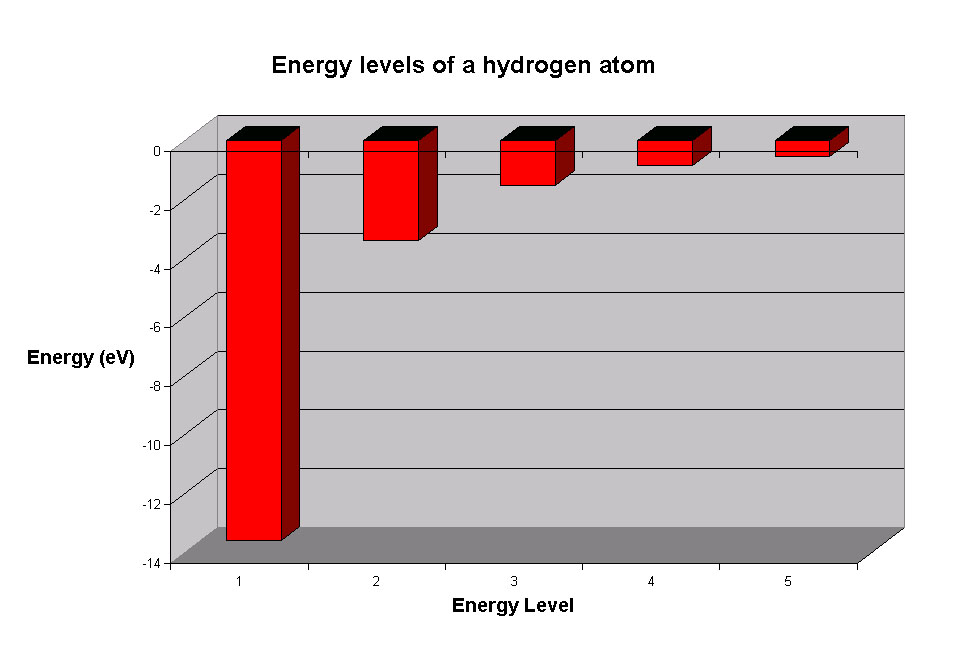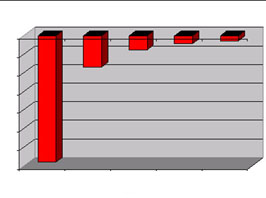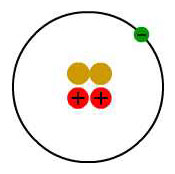Click to view animation.

As you may remember from chemistry, an atom consists of electrons orbiting around a nucleus. However, the electrons cannot choose any orbit they wish. They are restricted to orbits with only certain energies. Electrons can jump from one energy level to another, but they can never have orbits with energies other than the allowed energy levels.

Let’s look at the simplest atom, a neutral hydrogen atom. Its energy levels are given in the diagram below. The x-axis shows the allowed energy levels of electrons in a hydrogen atom, numbered from 1 to 5. The y-axis shows each level’s energy in electron volts (eV). One electron volt is the energy that an electron gains when it travels through a potential difference of one volt (1 eV = 1.6 x 10-19 Joules).Electrons in a hydrogen atom must be in one of the allowed energy levels. If an electron is in the first energy level, it must have exactly -13.6 eV of energy. If it is in the second energy level, it must have -3.4 eV of energy. An electron in a hydrogen atom cannot have -9 eV, -8 eV or any other value in between.

Let’s say the electron wants to jump from the first energy level, n = 1, to the second energy level n = 2. The second energy level has higher energy than the first, so to move from n = 1 to n = 2, the electron needs to gain energy. It needs to gain (-3.4) – (-13.6) = 10.2 eV of energy to make it up to the second energy level.

The electron can gain the energy it needs by absorbing light. If the electron jumps from the second energy level down to the first energy level, it must give off some energy by emitting light. The atom absorbs or emits light in discrete packets called photons, and each photon has a definite energy. Only a photon with an energy of exactly 10.2 eV can be absorbed or emitted when the electron jumps between the n = 1 and n = 2 energy levels.

The energy that a photon carries depends on its wavelength. Since the photons absorbed or emitted by electrons jumping between the n = 1 and n = 2 energy levels must have exactly 10.2 eV of energy, the light absorbed or emitted must have a definite wavelength. This wavelength can be found from the equation

`E = hc/l,`

where E is the energy of the photon (in eV), h is Planck’s constant (4.14 x 10-15 eV s) and c is the speed of light (3 x 108 m/s). Rearranging this equation to find the wavelength gives

`l = hc/E.`

A photon with an energy of 10.2 eV has a wavelength of 1.21 x 10-7 m, in the ultraviolet part of the spectrum. So when an electron wants to jump from n = 1 to n = 2, it must absorb a photon of ultraviolet light. When an electron drops from n = 2 to n = 1, it emits a photon of ultraviolet light.

The step from the second energy level to the third is much smaller. It takes only 1.89 eV of energy for this jump. It takes even less energy to jump from the third energy level to the fourth, and even less from the fourth to the fifth.

What would happen if the electron gained enough energy to make it all the way to 0eV? The electron would then be free of the hydrogen atom. The atom would be missing an electron, and would become a hydrogen ion.

The table below shows the first five energy levels of a hydrogen atom.Energy Level Energy
1 -13.6 eV
2 -3.4 eV
3 -1.51 eV
4 -.85 eV
5 -.54 eV
Exercise 2. Find the wavelength of a photon emitted when an electron jumps from the n = 3 energy level down to the n = 2 energy level. Where is this photon in the electromagnetic spectrum?
Exercise 3. The table below shows the energy levels of a singly ionized helium atom – an ion with two protons, two neutrons, and one electron:

Energy Level Energy
1 -54.4 eV
2 -13.6 eV
3 -6.04 eV
4 -3.4 eV
5 -2.176 eVHow much energy must be given off when the electron jumps from the second energy level down to the first energy level?

Exercise 4. What is wavelength of a photon emitted when an electron jumps from the n = 2 to n = 1 energy level of a singly ionized helium atom? Where is this photon in the electromagnetic spectrum?

You can use this method to find the wavelengths emitted by electrons jumping between energy levels in various elements. However, finding the correct energy levels gets much more difficult for larger atoms with many electrons. In fact, the energy levels of neutral helium are different from the energy levels of singly ionized helium! Therefore, we will skip how to calculate all the energy levels for different atoms for now. The energy levels are published in the CRC Handbook of Chemistry and Physics if you want to look them up.